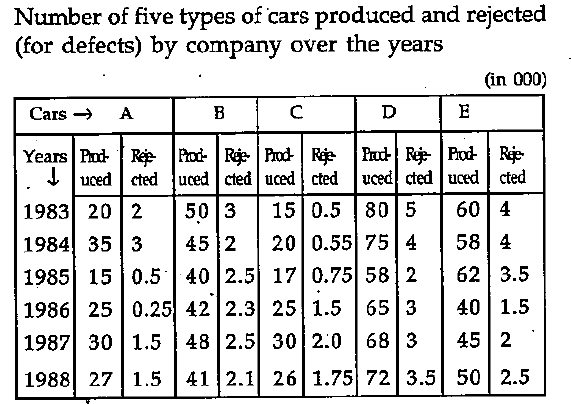# Data Interpretation - Tabular Data

> > > > > > > > Tabular Data

Instructions:

Study the following table carefully and answer the questions given below()

• A

1983• B

1884• C

1985• D

1887• Option : C
• Explanation : For B type car, the ratio of rejection to production in

1983 = 3/50 =0.060

1984 = 2/45 = 0.044

1985 = 2.5/40 = 0.063

1986 = 2.3/42 = 0.055

1987 = 2.5/48 = 0.052

1988 = 2.1/41 = 0.051

Instructions:

Study the following table carefully and answer the questions given below

()

• A

1984• B

1985• C

1986• D

1987• Option : C
• Explanation : For E type car, the ratio of rejection to production in 1983 = 0.067

Similar figure for other ahead years are 0.069; 0.056, 0.038, 0.044, 0.050 respectively

Instructions:

Study the following table carefully and answer the questions given below

()

• A

200• B

250• C

3000• D

2500• Option : D
• Explanation : (62-3.5) = k% of (58-4) k = (100*58.5)/54 = 108

Instructions:

Study the following table carefully and answer the questions given below

()

• A

A• B

B or E• C

B and C• D

None of these• Option : C
• Explanation : Rejection percent in 1984 for type A

= 300/35 = 8.57

and for other type cars the rejection percent is respectively 4.44 for type B; 2.75 for type C; 5.33 for type D; and 6.90 for type E

Instructions:

Study the following table carefully and answer the questions given below

()

The acceptable E type cars in 1985 was what percent to that of 1984?

• A

8• B

106• C

110• D

None of these## Description

• Tabular Data Analysis Questions can be used to give quizzes by any candidate who is preparing for UGC NET Paper 1
• This Tabular Data Analysis Questions section will help you test your analytical skills in a tricky method, thereby giving you an edge over other students
• All candidates who have to appear for the Kendriya Vidyalaya Entrance exam can also refer to this mcq section.
• You can also get access to the Tabular Data Analysis MCQ ebook.
• Tabular Data Analysis Questions can be used in the preparation of JRF, CSIR, and various other exams.